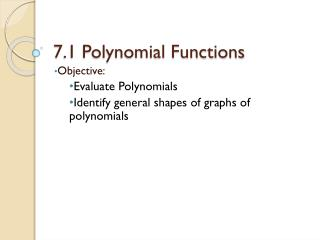Download Presentation7.1 Polynomial Functions

# 7.1 Polynomial Functions - PowerPoint PPT PresentationDownload Presentation## 7.1 Polynomial Functions

- - - - - - - - - - - - - - - - - - - - - - - - - - - E N D - - - - - - - - - - - - - - - - - - - - - - - - - - -
##### Presentation Transcript

1. 7.1 Polynomial Functions Objective: Evaluate Polynomials Identify general shapes of graphs of polynomials

2. Polynomial Function(in one variable) • degree of f(x): highest exponent • leading coefficient: the coefficient of the term with the highest degree

3. Examples: • State the degree & leading coefficient

4. Evaluate a Polynomial Function • Find f(6) • Find g(3)

5. Find f(a2) • Find f(h+3)

6. Graphs of Polynomial Functions • Constant Function degree = 0 =4 • Linear Function degree = 1

7. Graphs of Polynomial Functions • Quadratic Function degree = 2 =4 • Cubic Function degree = 3

8. Graphs of Polynomial Functions • Quartic Function degree = 4 • Quintic Function degree = 5

9. End Behavior of EVEN DEGREE • Leading Coefficient Positive • Leading Coefficient Negative

10. End Behavior of ODD DEGREE • Leading Coefficient Positive • Leading Coefficient Negative

11. Zeros (or Roots) The number of REAL ZEROS a polynomial can be determined by how many times the graph crosses the x-axis.

12. For each graph, - End Behavior?- Even or Odd?- # of Real Zeros? Even 1 Real Zeros Odd 3 Real Zeros Odd 3 Real Zeros